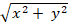Chapter 16.6, Problem 42E

Chapter
Section
Textbook Problem

Find the area of the surface.42. The part of the cone z =that lies between the plane y = x and the cylinder y = x2

To determine

To find: The area for surface of the part of the cone z=x2+y2 that lies between the plane y=x and the cylinder y=x2 .

Explanation

Given data:

The equation of the part of the cone is given as follows.

z=x2+y2

The cone lies between the plane y=x and the cylinder y=x2 .

Formula used:

Write the expression to find the surface area of the plane.

A(S)=D1+(zx)2+(zy)2dA (1)

Write the equation of part of the cone as follows.

z=x2+y2 (2)

Calculation of zx :

Take partial derivative for equation (2) with respect to x.

zx=xx2+y2=12x2+y2(2x)=xx2+y2

Calculation of zy :

Take partial derivative for equation (2) with respect to y.

zy=yx2+y2=12x2+y2(2y)=yx2+y2

Calculation of surface area of plane:

Substitute xx2+y2 for zx and yx2+y2 for zy in equation (1),

A(S)=D1+(xx2+y2)2+(yx2+y2)2dA=D1+x2x2+y2+y2x2+y2dA=D1+x2+y2x2+y2dA=D1+1dA

Simplify the expression as follows

Still sussing out bartleby?

Check out a sample textbook solution.

See a sample solution

The Solution to Your Study Problems

Bartleby provides explanations to thousands of textbook problems written by our experts, many with advanced degrees!

Get Started

Find more solutions based on key concepts